## Reciprocity Theorem

If there exists a Rational Integersuch that, when,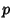, and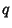are Positive Integers,thenis the-adic reside of, i.e.,is an-adic residue ofIffis solvable for. Reciprocity theorems relate statements of the formis an-adic residue of'' with reciprocal statements of the formis an-adic residue of.''

The first case to be considered was(the Quadratic Reciprocity Theorem), of which Gaußgave the first correct proof. Gauss also solved the case(Cubic Reciprocity Theorem) using Integers of the form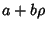, where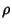is a root ofand,are rational Integers. Gaußstated the case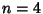(Quartic Reciprocity Theorem) using the Gaussian Integers.

Proof of-adic reciprocity for Primewas given by Eisenstein in 1844-50 and by Kummerin 1850-61. In the 1920s, Artin formulated Artin's Reciprocity Theorem, a general reciprocity law for all orders.

See also Artin Reciprocity, Cubic Reciprocity Theorem, Langlands Reciprocity, Quadratic Reciprocity Theorem, Quartic Reciprocity Theorem, Rook Reciprocity Theorem